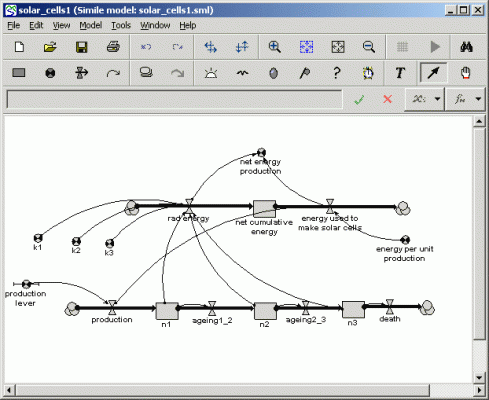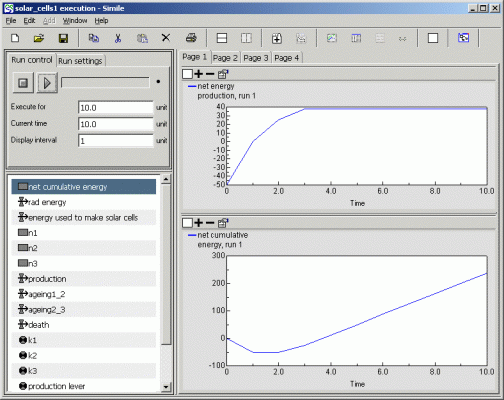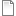# Solar cell energy payoff, version 1

ModelId:
solar_cells1
SimileVersion:
4+

## Introduction

The aim of this model is to assess the net benefit, in energy terms, of solar cell (photovoltaics) production.   The net benefit is the difference between amount of electricity generated and the amount of electricity need to manufacture the solar cells.

The idea of developing this model came from a report by Jennifer Leaf, entitled "DynSysMod: a gramework for modeling composite dynamic systems".  In the section "Energy System model" (p.23), the specification for a model is give, along with suggested ways of modelling the problem in Stella and DynSysMod.   The present solution is radically different from the two presented in that report.

The text description of the problem is given as:

"This model focuses on the conversion of solar energy to usable electricity and the uses of the generated electricity. The percentage of raw solar energy that can be converted to electricity is controlled by the overall efficiency of the solar cells, as well as the quality of the cells. The quality degrades over time, limiting the amount of energy that can be converted per unit time. A production process is in place to replace low quality solar cells, and it is assumed that adequate raw materials are available to support the production process.  However the production process consumes a percentage of the electricity generated by the conversion process, and generates waste material and heat."

## The model

The model has two parts: the energy flow part and the solar cell production part.

### Energy part

The energy part has a single compartment.   Unusually, this does not represent a physical store of some substance.   Rather, its job is simply an accounting one, to represent the total amount of useable energy accumulated during the course of the simulation.  This is the accumulated difference between energy production by the solar cells (capturing sunlight and converting this into electricity), and the energy needed for solar cell manufacture.

### Solar cell production part

As noted above, the quality of solar cells declines with time.  This suggests that it is necessary to divide the solar cells into age classes (just as one might do in a model of an animal population or trees in a forest).   In this first version of the model, this is done by assuming that the lifetime of solar cells is just 3 years, and having a compartment for each annual group (i.e. the number of solar cells up to 1, 2 and 3 years respectively).   The flow into the first class is production; the flows between the compartments is the ageing process; and the flow out of the thord class represents death of solar cells.

The calculation of energy production is simply the sum of the number of solar cells in each annual class multiplied by the efficiency (k) of cells in that class.   The energy loss is simply equal to the production of solar cells times the energy cost of producing each one.Results:AttachmentSizesolar_cells1.sml217.18 KBsolar_cells1_diagram.gif15.68 KBsolar_cells1_results.gif61.89 KB
Model tags: